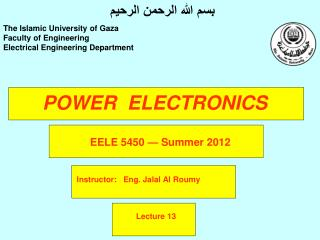DownloadDownload PresentationPOWER ELECTRONICS

# POWER ELECTRONICS

Download Presentation## POWER ELECTRONICS

- - - - - - - - - - - - - - - - - - - - - - - - - - - E N D - - - - - - - - - - - - - - - - - - - - - - - - - - -
##### Presentation Transcript

1. بسم الله الرحمن الرحيم The Islamic University of Gaza Faculty of Engineering Electrical Engineering Department POWER ELECTRONICS EELE 5450— Summer 2012 Instructor: Eng. Jalal Al Roumy Lecture 13

2. Chapter Five AC Line Voltage Control Phase-Control technique give the simplest ac regulating system and are the best known. • There are other methods by which thyristor control of ac lines is possible: • Zero voltage switching • Ac chopper control • synchronous tap changing Ac line voltage controllers are employed to vary the rms value of the alternating voltage applied to a load by using thyristors as a switch.

3. Chapter Five AC Line Voltage Control • The applications of ac line voltage controllers include the following: • Lighting controls • Induction heating • Industrial heating • Transformer tap changing • Speed control of induction motor

4. Chapter Five AC Phase Control With resistive Load This circuit provides a fixed half-cycle of power and a power control range of half-power minimum and full-power maximum, but with a strong dc component.

5. Chapter Five AC Phase Control With resistive Load This circuit is back-to-back SCRs which allows symmetrical control of ac voltage during both halves of the ac wave and eliminates and dc component.

6. Chapter Five AC Phase Control With resistive Load The most simple, efficient and reliable method of controlling ac power is by using the Triac. Triac is bidirectional device, and a single control circuit is sufficient to control power flow in the load during both halves of the ac input wave.

7. Chapter Five Analysis of Full-wave controller : resistive Load

8. Chapter Five Full-wave controller With Inductive Load In actual practice most loads have some amount of inductance. Motors, solenoids, transformers, and even some resistive heaters have inductive components as apart of their impedance. The effect of this reactance is that the rms-to-average-current ratio is lowered. Higher average current can be passed through the SCR. With an inductive load , current flow is maintained through the SCR even after the input voltage has reversed polarity and goes negative. The duration of this interval is determined bu the load power factor.

9. Chapter Five Full-wave controller With Inductive Load

10. Chapter Five Full-wave controller With Inductive Load

11. End of Lecture